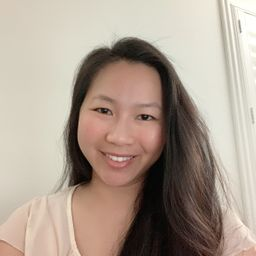# Geometry

## Educators### Problem 1

On your paper, draw two segments roughly like those shown. Use these segments in Exercises 1-4 to construct a segment having the indicated length.
$$a+b$$Amrita B.

### Problem 2

On your paper, draw two segments roughly like those shown. Use these segments in Exercises 1-4 to construct a segment having the indicated length.
$$b-a$$

Check back soon!

### Problem 3

On your paper, draw two segments roughly like those shown. Use these segments in Exercises 1-4 to construct a segment having the indicated length.
$$3 a-b$$Amrita B.

### Problem 4

On your paper, draw two segments roughly like those shown. Use these segments in Exercises 1-4 to construct a segment having the indicated length.
$$a+2 b$$

Check back soon!

### Problem 5

Using any convenient length for a side, construct an equilateral triangle.Amrita B.

### Problem 6

a. Construct a $30^{\circ}$ angle. $\qquad$ b. Construct a $15^{\circ}$ angle.

Check back soon!

### Problem 7

Draw any acute $\triangle A C U$ . Use a method based on the SSS Postulate to construct a triangle congruent to $\Delta A C U$ .Amrita B.

### Problem 8

Draw any obtuse $\triangle O B T$ . Use the SSS method to construct a triangle congruent to $\triangle O B T$ .Erik S.

### Problem 9

Repeat Exercise $7,$ but use the SAS method.

Check back soon!

### Problem 10

Repeat Exercise $8,$ but use the ASA method.

Check back soon!

### Problem 11

On your paper, draw two angles roughly like those shown. Then for Exercises $11-14$ construct an angle having the indicated measure.
$$x+y$$

Check back soon!

### Problem 12

On your paper, draw two angles roughly like those shown. Then for Exercises $11-14$ construct an angle having the indicated measure.
$$x-y$$Tiffany T.

### Problem 13

On your paper, draw two angles roughly like those shown. Then for Exercises $11-14$ construct an angle having the indicated measure.
$$\frac{3}{4} x$$

Check back soon!

### Problem 14

On your paper, draw two angles roughly like those shown. Then for Exercises $11-14$ construct an angle having the indicated measure.
$$180-2 y$$

Check back soon!

### Problem 15

a. Draw any acute triangle. Bisect each of the three angles.
b. Draw any obtuse triangle. Bisect each of the three angles.
c. What do you notice about the points of intersection of the bisectors in parts ( a ) and (b)?Amrita B.

### Problem 16

Construct a six-pointed star using the following procedure.
1. Draw a ray, $\overrightarrow{A B}$ . On $\overrightarrow{A B}$ mark off, in order, points $C$ and $D$ such that $A B=B C=C D$ .
2. Construct equilateral $\triangle A D G$ .
3. On $\overline{A G}$ mark off points $E$ and $F$ so that both $A E$ and $E F$ equal $A B$ .
4. On $\overline{G D}$ mark off points $H$ and $I$ so that both $G H$ and $H I$ equal $A B .$
5. To complete the star, draw the three lines $\stackrel{\leftrightarrow}{F H}, \stackrel{\leftrightarrow}{E B},$ and $\stackrel{\leftrightarrow}{C l}$ .

Check back soon!

### Problem 17

Construct an angle having the indicated measure.
$$120$$Amrita B.

### Problem 18

Construct an angle having the indicated measure.
$$150$$

Check back soon!

### Problem 19

Construct an angle having the indicated measure.
$$165$$Amrita B.

### Problem 20

Construct an angle having the indicated measure.
$$45$$

Check back soon!

### Problem 21

Draw any $\Delta A B C$ . Construct $\triangle D E F$ so that $\triangle D E F \sim \triangle A B C$ and $D E=2 A B .$Amrita B.

### Problem 22

Construct a $\triangle R S T$ such that $R S : S T : T R=4 : 6 : 7$

Check back soon!

### Problem 23

On your paper draw figures roughly like those shown. Use them in constructing the figures described in Exercises 23-25.
An isosceles triangle with a vertex angle of $n^{\circ}$ and legs of length $d$

Check back soon!

### Problem 24

On your paper draw figures roughly like those shown. Use them in constructing the figures described in Exercises 23-25.
An isosceles triangle with a vertex angle of $n^{\circ}$ and base of length $s$

Check back soon!

### Problem 25

On your paper draw figures roughly like those shown. Use them in constructing the figures described in Exercises 23-25.
A parallelogram with an $n^{\circ}$ angle. longer side of length $s,$ and longer diagonal of length $d$

Check back soon!

### Problem 26

On your paper draw figures roughly like the ones shown. Then construct a triangle whose three angles are congruent to $\angle 1 . \angle 2$ , and $\angle 3$ , and whose circumscribed circle has radius $r$ .

Check back soon!